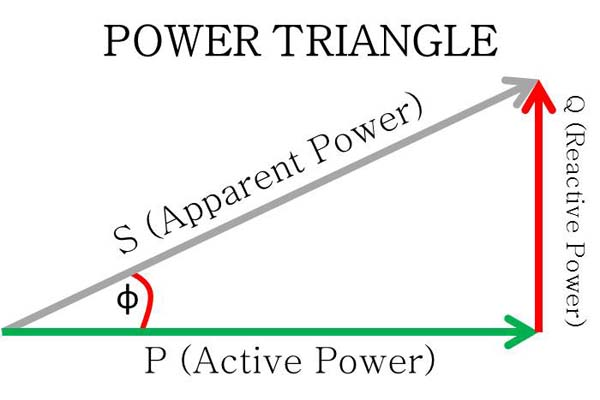# Explanation of Power Triangle and Three Types of Power

Explanation about what is Power Triangle and Three Types of Power?, In our daily lives we certainly often hear the word of power that is in Watt units, Like for example the electrical power installed in our home installations, some have 900 Watt power, 1300 Watt, 2200 Watt and so on, or on Any Electrical Appliances, like Electric Iron 300 Watts, Light Bulb 45 Watts, Fan 75 Watts, Air conditioner 350 Watt, and etc.

Electricity that has Single Phase, only has one type of electric power, namely Active power in Watts unit, But it is different in Three Phase Electricity there are 3 (three) Types of Power, Namely:
• P = Active, Real or True Power in Watt (W) or Kilowatts (KW) Units
• S = Apparent Power in Voltampere (Va) or Kilovolt Ampere(KVA) Units
• Q = Reactive Power in Voltampere Reactive (VAr) or KiloVoltAmpere Reactive (KVAR)
And these three types of power are related to one another, and can be described by the Power triangle

## Explanation of Power Triangle and Three Types of PowerPower Triangle and Three Types of Power
This happens because the 3 Phase electrical installation has various factors that can cause power losses, The Power Factor in a Single Phase electrical installation has a value of 1.00, which is why Apparent Power in single phase electricity is the same as Active Power.
Active Power (W) = Apparent Power (VA), if Cosphi is One

Because the Cosphi Value for electricity in single Phase is One, the only available Power is Active Power (Watt).

Power Factor or Cosphi in Three Phase Electricity is Different with in Single Phase, 3 Phase electricity has a Power factor or Cosphi is below One, Then:
Active Power = Apparent Power x Cosphi
From the formula above, we can see that if the Cosphi value approaches 1.00, the greater Active Power that we can use.

The thing that affects the high and low values of Cosphi is how much Reactive Power is generated in the 3-phase electrical installations, and Reactive power is generated from how much electrical equipment that produces harmonic power used, this Harmonic power is what causes power losses.

Explanation of Three Types of Power

Apparent Power (S)
In 3 Phase electrical installations, we know there are Three Types of Power, one of which is Apparent Power, Apparent power Is the power that generated from electricity calculations before using to electrical loads.

Apparent Power (VA) is produced from the calculation results of the formula, that is, Apparent Power (S) equal to Voltage (V) multiplied to Current (A).
S = V x I x √3
Apparent-power is also known as Total Power, or the power written on the Nameplate of an electric appliance or at A Generator.

Active Power (P)
In 3 (three) Phase electrical installations, the second type of power is Active Power, Active Power Is the actual power that we can use and usually the active power is lower than the apparent power, Active Power is the results of Calculation of Apparent Power multiplied with a power factor (Cosphi).
P = S x Cosphi x √3
Active Power will experience a decrease in value due to electrical loads that produce reactive power, Examples of loads / electrical devices that produce reactive power / harmony power.

Electrical equipment that produces Harmonic Power:
• Electro Motor
• Electric welding Machine
• Transformer
• Inverter
• Variable speed Drives
• Fluorescent Lighting
• UPS

Reactive power (Q)
The next power contained in a 3 Phase electrical installation is Reactive Power, Reactive Power Is the power that causes power losses, or power that results in a decrease in the value of the power factor (Cosphi), Value of the reactive power depends on how many electrical devices that produce Reactive power.

Power factor (Cosphi)
Power factor is the ratio between active power (W) and apparent power (VA), Or cosine angle between active power and apparent power (see figure of Power Triangle above).

High reactive power will cause a greater cosphi angle, and consequently the power factor will be lower, and Power factor is always smaller or equal to one.

In calculation, if the Cosphi value is 1.00. then the value of Active Power will be the same as the Apparent Power value, Low power factor will result in various power losses, because it will result in high load currents, The usual way to improve the low power factor is to install a Capacitor Bank in the 3 Phase Electrical Installation.

Example calculation of Three Phase electrical installation systems that have the same voltage and current but have different Power factors, then it will have different Active Power.

Example 1
Three Phase Electrical System that has a voltage of 400 volts and a current of 200 amperes, cosphi 0.95, Then:
• S (Apparent Power) = V x I x √3
• S (Apparent Power) = 400 x 200 x 1.73
• S (Apparent Power) = 138,400 VA
• P (Active Power) = V x I x Cosphi x √3
• P (Active Power) = 400 x 200 x 0.95 x 1.73
• P (Active Power) = 131,480 Watt

Example 2
Three Phase Electrical System that has a voltage of 400 volts and a current of 200 amperes, cosphi 0.85, Then:
• S (Apparent Power) = V x I x √3
• S (Apparent Power) = 400 x 200 x 1.73
• S (Apparent Power) = 138,400 VA
• P (Active Power) = V x I x Cosphi x √3
• P (Active Power) = 400 x 200 x 0.85 x 1.73
• P (Active Power) = 117,640 Watt
The lower the value of the power factor (Cosphi) the lower value of the active power generated by an electrical installation

My Electrical Diary